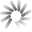\$10,985.72 +0.09 %
\$375.04 -1.76 %
\$92.38 -0.68 %
\$71.35 -0.72 %
\$57.81 +0.10 %
\$47.15 -2.35 %# Unobtanium Hashrate Chart

The Unobtanium hashrate chart provides the current Unobtanium hashrate history in graph format with an option to expand the Unobtanium global hashrate chart time frame back to 2015.

##Loading Unobtanium network hashrate chart

The Unobtanium network hashrate chart can be used to visualize Unobtanium mining hashrate increases and decreases viewable in segment options of daily, weekly, monthly, 3 months, 6 months, 1 year, 3 years, and all time.

## What is Unobtanium Hashrate?

Unobtanium hashrate is a calculated numerical value that specifies an estimate of how many hashes are being generated by Unobtanium miners trying to solve the current Unobtanium block or any given block.

Unobtanium hashrate is represented in Hashes per Second or H/s.

The global Unobtanium network hashrate is a calculated value and is measured in hashes per second (H/s). The calculation uses the current mining difficulty and the average Unobtanium block time between mined blocks versus the defined block time as variables to determine the global Unobtanium network hashrate.

As the Unobtanium network hashrate goes up - the UNO hashrate numbers get so large that abbreviations must be used.

The abbreviations are SI derived units representing the number of hashes performed in a one second time frame.

### The current Unobtanium hashrate is 12.51 EH/s, representing the global Unobtanium network hashrate with a mining difficulty of 585.75 G at block height 1,532,119.

Hashrate Unit/s Hash Hashes Per Second
H/s (Hash) 1 One
kH/s (KiloHash) 1,000 One Thousand
MH/s (MegaHash) 1,000,000 One Million
GH/s (GigaHash) 1,000,000,000 One Billion
TH/s (TeraHash) 1,000,000,000,000 One Trillion
EH/s (ExaHash) 1,000,000,000,000,000,000 One Quintillion
ZH/s (ZettaHash) 1,000,000,000,000,000,000,000 One Sextillion
YH/s (YottaHash) 1,000,000,000,000,000,000,000,000 One Septillion

It is important to point out the Unobtanium hashrate does not determine how quickly or slowly each block is solved.

This timing, called the block time is enforced by the Unobtanium mining difficulty value, which is adjusted upwards or downwards during each block difficulty retarget to keep blocks being solved at a constant time frame.

You can calculate Unobtanium mining profits using the current UNO hashrate difficulty and our Unobtanium hashrate calculator.

## What is the Current Unobtanium Hashrate?

The current Unobtanium hashrate (UNO hashrate) is 12.51 EH/s at block height 1,532,119 with a difficulty of 585,748,963,763.25.

Unobtanium Hashrate Stats

### Current Unobtanium Hashrate

Unobtanium Global Hashrate
12.51 EH/s

### Unobtanium Hashrate All Time High

Unobtanium Hashrate on Jan 23, 2020 at block 1,416,898
18.09 EH/s

## How to Calculate Unobtanium Hashrate

The Unobtanium hashrate is calculated using the current Unobtanium difficulty, the defined Unobtanium block time, and the average block time of the last (X) number of blocks.

Most full Unobtanium nodes will have an option to see the current Unobtanium global hashrate using the "getnetworkhashps" command in the console window.Given the fact that the Unobtanium difficulty value determines statically how many hashes are needed to solve the next block, it is recommended to use the current mining difficulty to determine the statistically accurate number of Unobtanium that can be mined in a given time frame.

Once again we recommend using our Unobtanium hashrate difficulty calculator as the current Unobtanium difficulty is preloaded, along with the latest Unobtanium price.

N/A
\$0.00 (0.00 %)

24 hour change